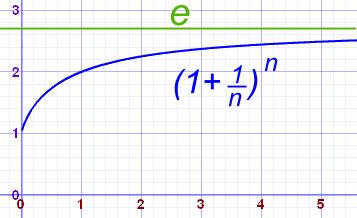Definition of

# e (Euler`s Number)The number "e" is one of the most important numbers in mathematics.

The first few digits are:

2.7182818284590452353602874713527... (and more)

It is often called Euler's number after Leonhard Euler.

It is the base of the natural logarithm.

It can be calculated many ways, for example the value of (1 + 1/n)n approaches e as n gets bigger and bigger: